Courses

# Chapter 9 State Variable Approach - Notes, Control System, Electrical Engineering Electrical Engineering (EE) Notes | EduRev

## Electrical Engineering (EE) : Chapter 9 State Variable Approach - Notes, Control System, Electrical Engineering Electrical Engineering (EE) Notes | EduRev

The document Chapter 9 State Variable Approach - Notes, Control System, Electrical Engineering Electrical Engineering (EE) Notes | EduRev is a part of the Electrical Engineering (EE) Course Electrical Engineering SSC JE (Technical).
All you need of Electrical Engineering (EE) at this link: Electrical Engineering (EE)

State Variable Approach

• A mathematical abstraction to represent or model the dynamics of a system utilizes three types of variables called the input, the output and the state variable.
• The state of a dynamical system is a minimal set of variables (known as state variables) such that the knowledge of these variables at t = t0 together with the knowledge of the inputs for ≥ t0 , completely determines the behaviour of the system for t > t0.

State Model

X = AX + BU State equations

Y = CX + DU Output equations

And both equations combined together is called state model.
X - State vector

U - Input vector

Y - Output Vector

A - System matrix

B - Input matrix

C - Output matrix

D - Transmission matrix

• It is applicable for both linear time-invariant and linear time-variant systems.
• It takes initial conditions into account.
• All the internal states of the system can be determined
• Applicable for multiple input multiple output
• Controllability and observability can be determined easily

REPRESENTATION OF STATE MODEL

• Physical variable representation.
• Phase variable representation.
• Canonical representation.

Note: State model of a system is not unique property but transfer function of the system is unique.

Physical Variable Representation

• Variables like current, voltage, velocity, distance etc. are taken as state variable.
• In electrical systems, current through each inductor and voltage across each capacitor is taken as state variable.

Example: Find out state model for the network shown below: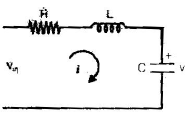Solution: Applying KVL,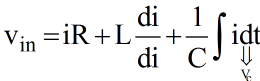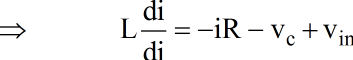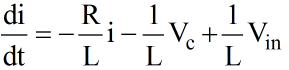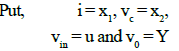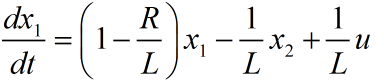........(i)

= f(x1, x2, u)

From n/w,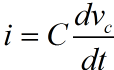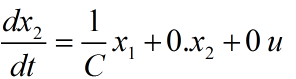Also, Voutput = Vo

Y = x2
= (0)x1 + (1)x2 + (0) u ...(ii)

writing equation (i), (ii), (iii) in matrix form,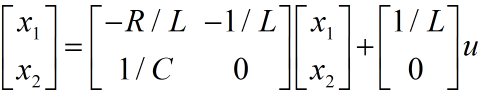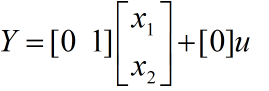Phase Variable Representation

• Take output and its derivative as the state variable this representation is used for the systems when can be represented by a linear differential equation.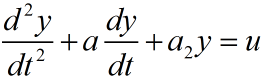Put y = x1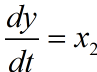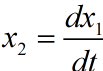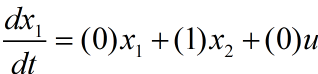From equation we have,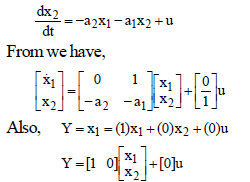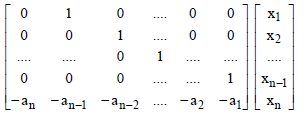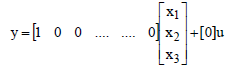Canonical Representation

X = A'X + B'u

Y = C'X + D'u

A' →  Diagonal matrix

Note:

• Diagonal elements represent the eigen values of the system matrix.
• Poles of the system are same as the eigen values of system matrix therefore in canonical representation the diagonal elements of system matrix represents pole of the system. 

Transfer function from state model

X = AX + BU
Y = CX + DU

Y → single output

U → single input

Initial conditions X(0) = 0 (for transfer function)

Taking Laplace of equation

sX(s) = AX)s) + BU(s)

sX(s) – AX(s) = BU(s)

[sI – A] = BU(s)

Taking Laplace of equation

Y(s) = CX(s) + DU(s)

Putting expression of X(s) from we have,

Y(s) = {C[sI – A]–1 B + D} U (s)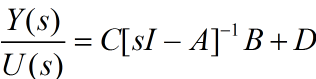SOLUTION OF STATE EQUATIONS

(i) Homogeneous System (U = 0) :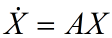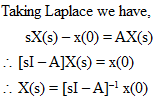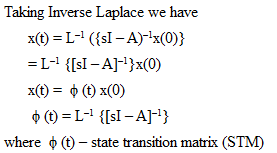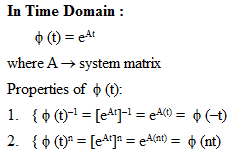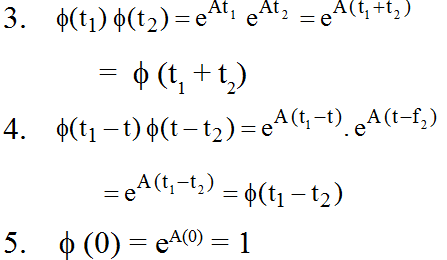(ii) Non-homogeneous System (U ≠ 0):

X = AX + BU

Taking Laplace transform we have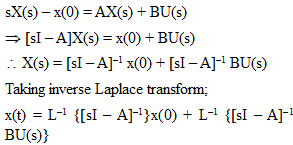Solution of State Equation in Time Domain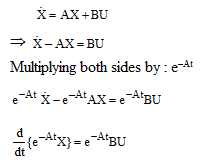Integrating both both sides we get,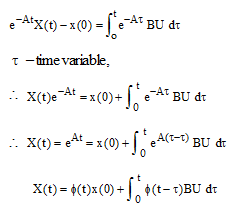CONTROLLABILITY & OBSERVABILITY

Definition of Controllability 

• A system is said to be controllable the state of the system can be transferred to another desired state over a given time period by using input

Kalman’s Test for Controllability

Consider,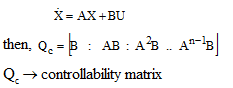According to Kalman’s Test

System is completely controllable if rank of matrix Qc is equal to order of the system i.e. (r = n) 

System will not be completely controllable (uncontrollable) if rank of matrix Qc is less than order of the system i.e. (r < n)

then number of uncontrollable states = (n – r)

number of controllable states = r

Rank of matrix Qc = n i.e. r = n

if det.Qc ≠ 0

Observability

• A system is said to be observable if all the states of the system can be determined on the basis of knowledge of the output of the system at a given time.

Kalman's Test for Observability

Q0 =[CT AT CT (AT)2CT....(A)n-1 CT]

n → order of system

Q0 → Observability testing matrix

X = AX + BU

Y = CX + DU

• System will be completely observable if rank of Q0 i.e r = n (order system) 
• System will be  unobservable if rank of r < n

Number of unobservable states = n – r

Number of observable states = r

Note: r = n if |Q0| ≠ 0

Duality 

• If AB is controllable then ATBT observable.
• If AC is observable then ATCT controllable.

JORDAN MATRIX

• If eigen values of system matrix A are repeated then in canonical representation the system matrix A' is not a diagonal matrix but a matrix called Jordan matrix.
• The diagonal elements of Jordan matrix represents eigen values of the system matrix.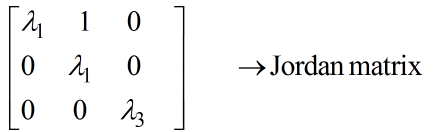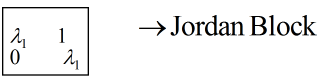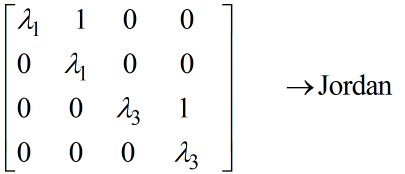Matrix =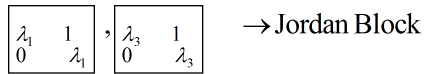Offer running on EduRev: Apply code STAYHOME200 to get INR 200 off on our premium plan EduRev Infinity!

65 docs|37 tests

,

,

,

,

,

,

,

,

,

,

,

,

,

,

,

,

,

,

,

,

,

,

,

,

,

,

,

;